# Section 5 3 NonHomogeneous Equations Homogeneous Linear Equations

• Slides: 13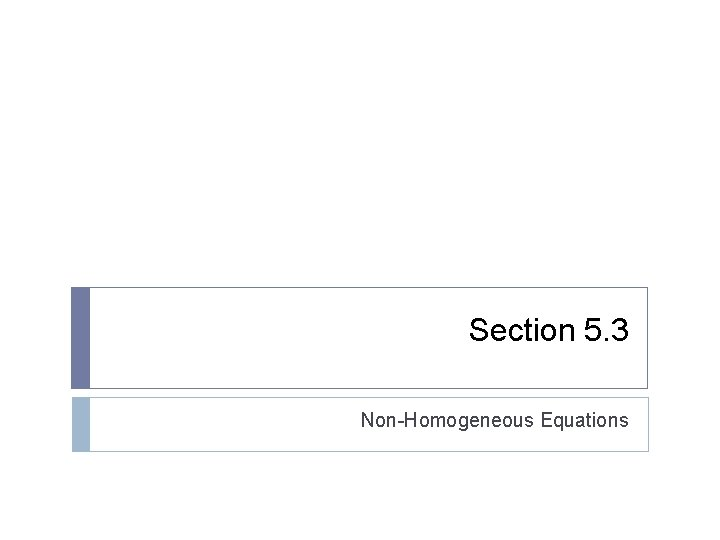Section 5. 3 Non-Homogeneous EquationsHomogeneous Linear Equations � Linearly independent solutions (guaranteed 2) � General solutions (with 2 constants) � Particular solutions (with 2 IC) � Wronskian for linear independence � Characteristic equation for linear equations with constant coefficients Simple real roots � Repeated real roots �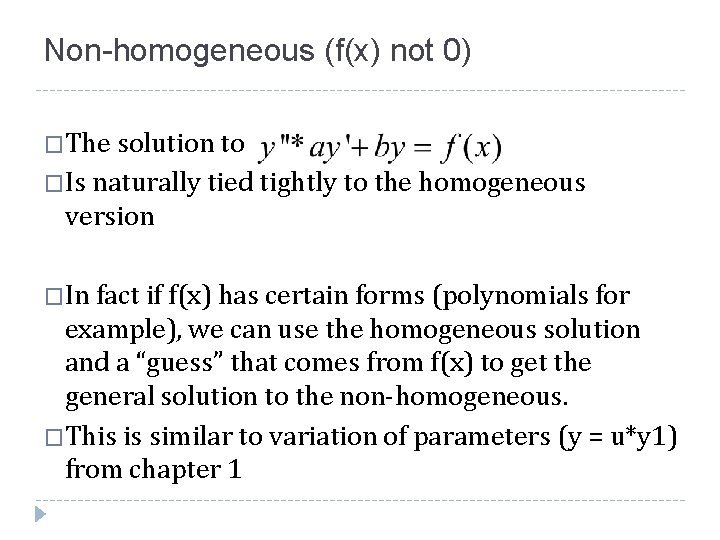Non-homogeneous (f(x) not 0) �The solution to �Is naturally tied tightly to the homogeneous version �In fact if f(x) has certain forms (polynomials for example), we can use the homogeneous solution and a “guess” that comes from f(x) to get the general solution to the non-homogeneous. �This is similar to variation of parameters (y = u*y 1) from chapter 1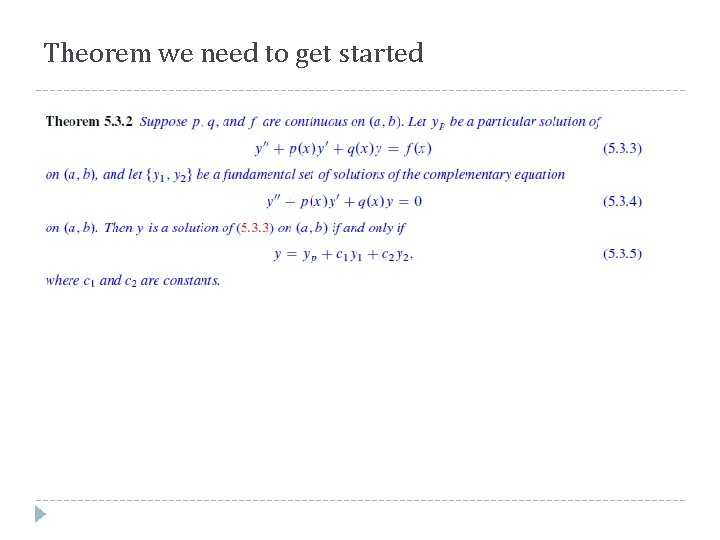Theorem we need to get started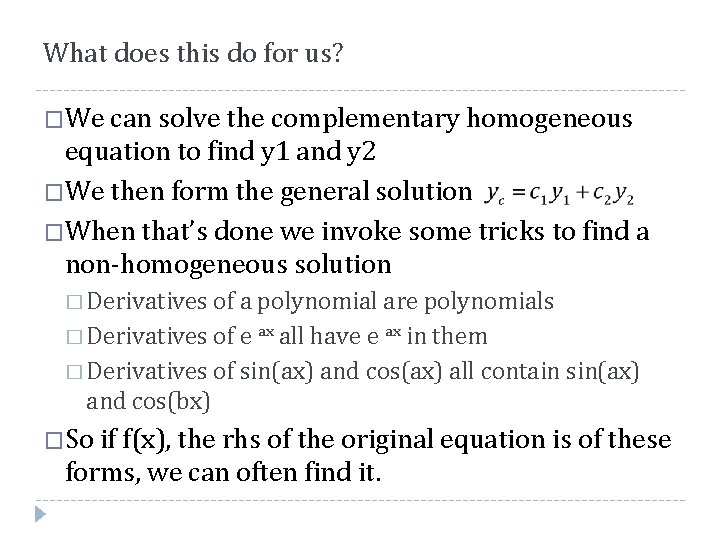What does this do for us? �We can solve the complementary homogeneous equation to find y 1 and y 2 �We then form the general solution �When that’s done we invoke some tricks to find a non-homogeneous solution � Derivatives of a polynomial are polynomials � Derivatives of e ax all have e ax in them � Derivatives of sin(ax) and cos(ax) all contain sin(ax) and cos(bx) �So if f(x), the rhs of the original equation is of these forms, we can often find it.Let’s take an example, and identify all of the notation � It is customary to denote the solution to the complementary as yc, then carry that around as needed � Let’s set up the complementary homogeneous equation and find its general solution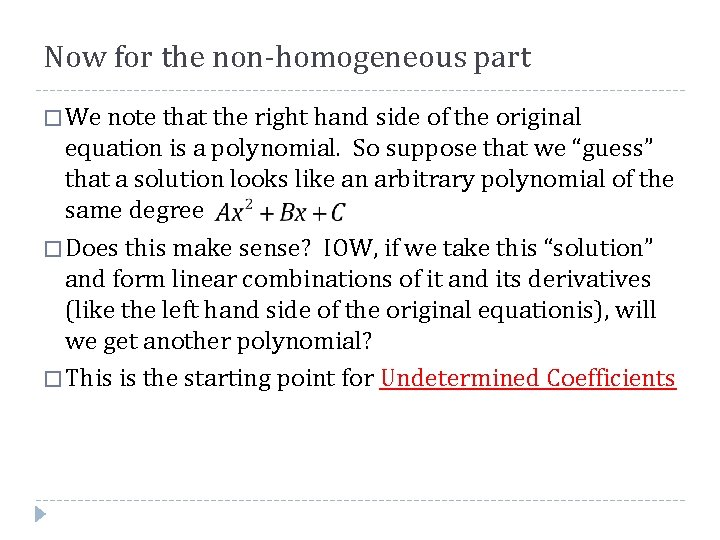Now for the non-homogeneous part � We note that the right hand side of the original equation is a polynomial. So suppose that we “guess” that a solution looks like an arbitrary polynomial of the same degree � Does this make sense? IOW, if we take this “solution” and form linear combinations of it and its derivatives (like the left hand side of the original equationis), will we get another polynomial? � This is the starting point for Undetermined Coefficients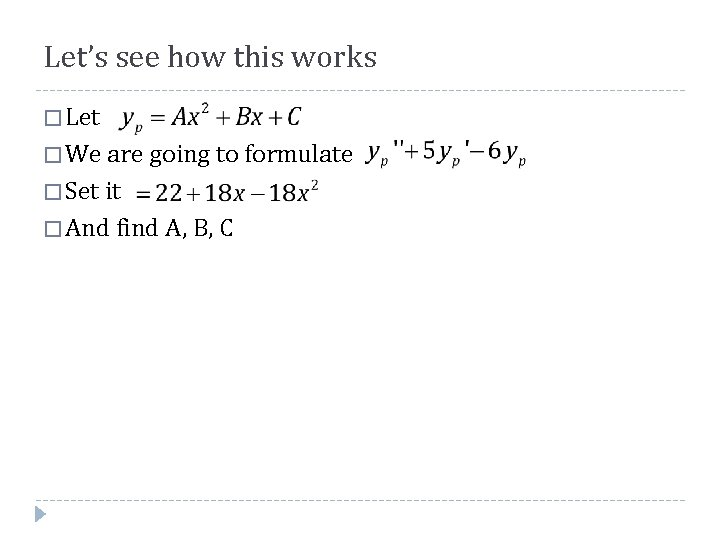Let’s see how this works � Let � We are going to formulate � Set it � And find A, B, C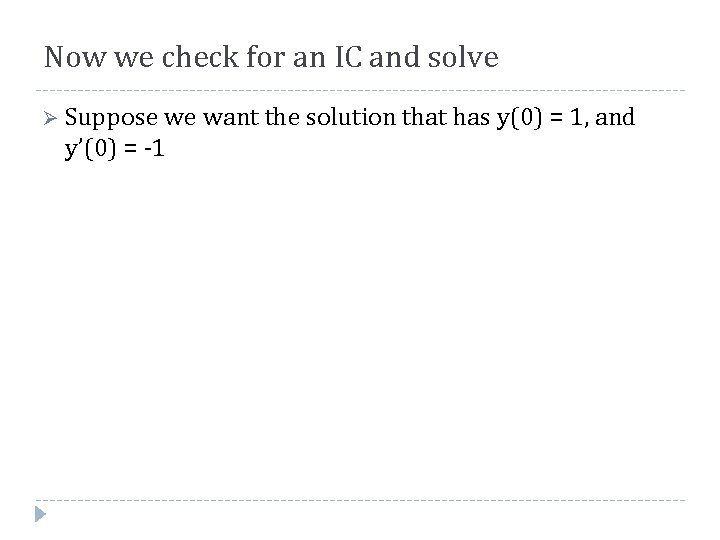Now we check for an IC and solve Ø Suppose we want the solution that has y(0) = 1, and y’(0) = -1Now for the second part, we change f(x) � We already saw that because the derivatives of polynomials are polynomials, we can solve for a particular solution if f(x) is a polynomial � On the other hand, all of the linear combinations of derivatives of are also linear combinations of � So in the equation � If f(x) consists of a single exponential term, we can “guess” at a solution and determine the coefficients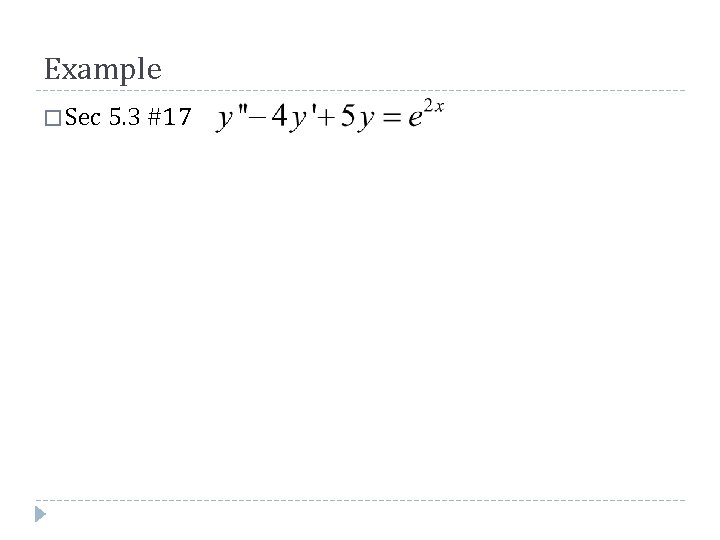Example � Sec 5. 3 #17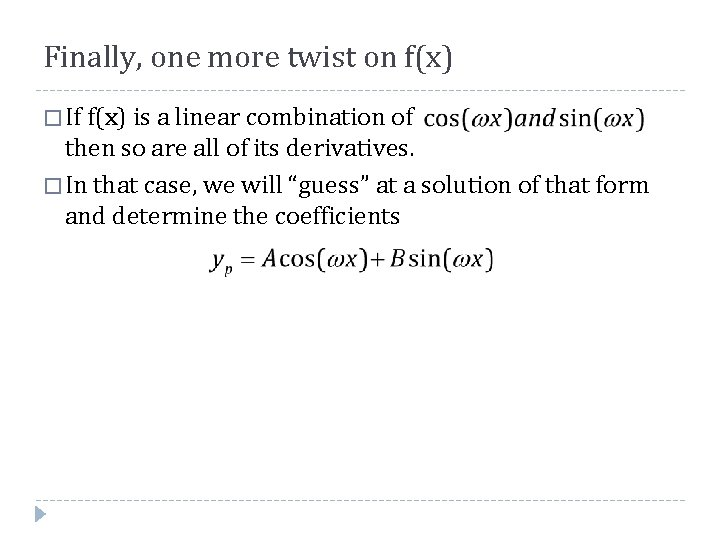Finally, one more twist on f(x) � If f(x) is a linear combination of then so are all of its derivatives. � In that case, we will “guess” at a solution of that form and determine the coefficients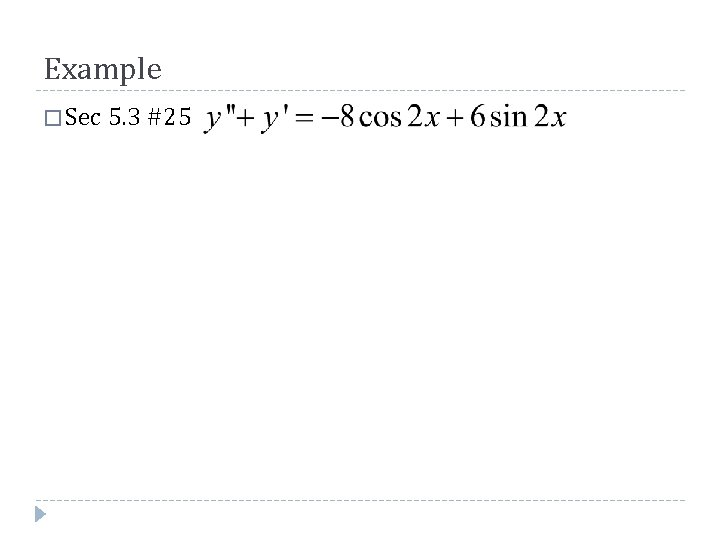Example � Sec 5. 3 #25